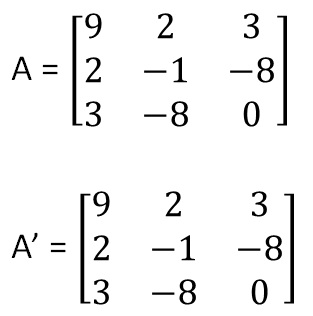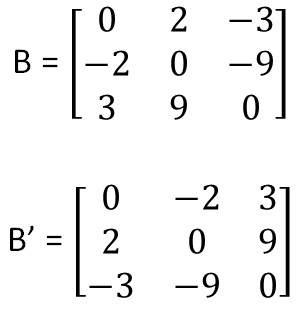Symmetric and skew symmetric matrices

Chapter 3 Class 12 Matrices
Concept wise

In a symmetric matrix,

A’ = A

And in a skew symmetric matrix

A’ = −A

Note: Here matrix should be a square matrix

Let’s take some examples

ForSince A = A’

∴ A is a symmetric matrix

ForTherefore,

B’ = −B

So, B is a skew symmetric matrix

Note: In a skew symmetric matrix, diagonal elements are always 0.

Learn in your speed, with individual attention - Teachoo Maths 1-on-1 Class